# Schelling's segregation model

In this introductory example we parallelize the main Tutorial while building the following definition of Schelling's segregation model:

• Agents belong to one of two groups (0 or 1).
• The agents live in a two-dimensional grid. Only one agent per position is allowed.
• For each agent we care about finding all of its 8 nearest neighbors (cardinal and diagonal directions). To do this, we will create a GridSpaceSingle with a Chebyshev metric, and when searching for nearby agents we will use a radius of 1 (which is also the default). This leads to 8 neighboring positions per position (except at the edges of the grid).
• If an agent has at least k=3 neighbors belonging to the same group, then it is happy.
• If an agent is unhappy, it keeps moving to new locations until it is happy, while respecting the 1-agent-per-position rule.

Schelling's model shows that even small preferences of agents to have neighbors belonging to the same group (e.g. preferring that at least 3/8 of neighbors to be in the same group) could still lead to total segregation of neighborhoods.

This model is also available as Models.schelling.

## Creating a space

using Agents

space = GridSpaceSingle((10, 10); periodic = false)
GridSpaceSingle with size (10, 10), metric=chebyshev, periodic=false

Notice that by default the GridSpaceSingle has metric = Chebyshev(), which is what we want. Agents existing in this type of space must have a position field that is a NTuple{2, Int}. We ensure this below by using @agent along with the minimal agent for grid spaces, GridAgent{2}.

## Defining the agent type

@agent SchellingAgent GridAgent{2} begin
mood::Bool # whether the agent is happy in its position. (true = happy)
group::Int # The group of the agent, determines mood as it interacts with neighbors
end

We added two more fields for this model, namely a mood field which will store true for a happy agent and false for an unhappy one, and an group field which stores 0 or 1 representing two groups.

Do notice that GridAgent{2} attributed the id::Int field, and the pos::NTuple{2, Int} field to our agent type

for (name, type) in zip(fieldnames(SchellingAgent), fieldtypes(SchellingAgent))
println(name, "::", type)
end
id::Int64
pos::Tuple{Int64, Int64}
mood::Bool
group::Int64

All these fields can be accessed during the simulation, but it is important to keep in mind that id must never be modified, and pos must be modified only through valid API functions such as move_agent!.

The @agent macro defined the following expression:

mutable struct SchellingAgent <: AbstractAgent
id::Int             # The identifier number of the agent
pos::NTuple{2, Int} # The x, y location of the agent on a 2D grid
mood::Bool          # ...
group::Int          # ...
end

## Creating an ABM

To make our model we follow the instructions of AgentBasedModel. We also want to include a property min_to_be_happy in our model, and so we have:

properties = Dict(:min_to_be_happy => 3)
schelling = ABM(SchellingAgent, space; properties)
AgentBasedModel with 0 agents of type SchellingAgent
space: GridSpaceSingle with size (10, 10), metric=chebyshev, periodic=false
scheduler: fastest
properties: min_to_be_happy

Here we used the default scheduler (which is also the fastest one) to create the model. We could instead try to activate the agents according to their property :group, so that all agents of group 1 act first. We would then use the scheduler Schedulers.ByProperty like so:

schelling2 = ABM(
SchellingAgent,
space;
properties = properties,
scheduler = Schedulers.ByProperty(:group),
)
AgentBasedModel with 0 agents of type SchellingAgent
space: GridSpaceSingle with size (10, 10), metric=chebyshev, periodic=false
scheduler: Agents.Schedulers.ByProperty{Symbol}
properties: min_to_be_happy

Notice that Schedulers.ByProperty accepts an argument and returns a struct, which is why we didn't just give Schedulers.ByProperty to scheduler.

## Creating the ABM through a function

Here we put the model instantiation in a function so that it will be easy to recreate the model and change its parameters.

In addition, inside this function, we populate the model with some agents. We also change the scheduler to Schedulers.Randomly. Because the function is defined based on keywords, it will be of further use in paramscan below.

using Random # for reproducibility
function initialize(; numagents = 320, griddims = (20, 20), min_to_be_happy = 3, seed = 125)
space = GridSpaceSingle(griddims, periodic = false)
properties = Dict(:min_to_be_happy => min_to_be_happy)
rng = Random.MersenneTwister(seed)
model = ABM(
SchellingAgent, space;
properties, rng, scheduler = Schedulers.Randomly()
)

# populate the model with agents, adding equal amount of the two types of agents
# at random positions in the model
for n in 1:numagents
agent = SchellingAgent(n, (1, 1), false, n < numagents / 2 ? 1 : 2)
end
return model
end
initialize (generic function with 1 method)

Notice that the position that an agent is initialized does not matter in this example. This is because we use add_agent_single!, which places the agent in a random, empty location on the grid, thus updating its position.

## Defining a step function

Finally, we define a step function to determine what happens to an agent when activated. For the purpose of this implementation of Schelling's segregation model, we only need an agent step function and not a model stepping function.

function agent_step!(agent, model)
minhappy = model.min_to_be_happy
count_neighbors_same_group = 0
# For each neighbor, get group and compare to current agent's group
# and increment count_neighbors_same_group as appropriately.
# Here nearby_agents (with default arguments) will provide an iterator
# over the nearby agents one grid point away, which are at most 8.
for neighbor in nearby_agents(agent, model)
if agent.group == neighbor.group
count_neighbors_same_group += 1
end
end
# After counting the neighbors, decide whether or not to move the agent.
# If count_neighbors_same_group is at least the min_to_be_happy, set the
# mood to true. Otherwise, move the agent to a random position, and set
# mood to false.
if count_neighbors_same_group ≥ minhappy
agent.mood = true
else
agent.mood = false
move_agent_single!(agent, model)
end
return
end
agent_step! (generic function with 1 method)

When defining agent_step!, we used some of the built-in functions of Agents.jl, such as nearby_positions that returns the neighboring position on which the agent resides, ids_in_position that returns the IDs of the agents on a given position, and move_agent_single! which moves agents to random empty position on the grid. A full list of built-in functions and their explanations are available in the API page.

## Stepping the model

Let's initialize the model with 370 agents on a 20 by 20 grid.

model = initialize()
AgentBasedModel with 320 agents of type SchellingAgent
space: GridSpaceSingle with size (20, 20), metric=chebyshev, periodic=false
scheduler: Agents.Schedulers.Randomly
properties: min_to_be_happy

We can advance the model one step

step!(model, agent_step!)

Or for three steps

step!(model, agent_step!, 3)

## Visualizing the data

There is a dedicated tutorial for visualization, animation, and interaction for agent based models. See Visualizations and Animations for Agent Based Models.

We can use the abmplot function to plot the distribution of agents on a 2D grid at every generation, via the InteractiveDynamics.jl package and the Makie.jl plotting ecosystem.

Let's color the two groups orange and blue and make one a square and the other a circle.

using InteractiveDynamics
using CairoMakie # choosing a plotting backend

groupcolor(a) = a.group == 1 ? :blue : :orange
groupmarker(a) = a.group == 1 ? :circle : :rect
figure, _ = abmplot(model; ac = groupcolor, am = groupmarker, as = 10)
figure # returning the figure displays it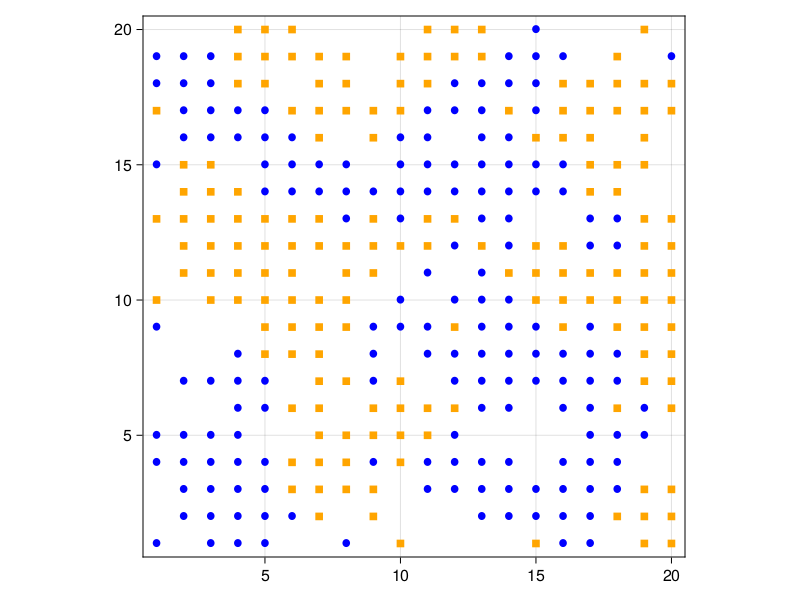## Animating the evolution

The function abmvideo can be used to save an animation of the ABM into a video. You could of course also explicitly use abmplot in a record loop for finer control over additional plot elements.

model = initialize();
abmvideo(
"schelling.mp4", model, agent_step!;
ac = groupcolor, am = groupmarker, as = 10,
framerate = 4, frames = 20,
title = "Schelling's segregation model"
)

## Collecting data during time evolution

We can use the run! function with keywords to run the model for multiple steps and collect values of our desired fields from every agent and put these data in a DataFrame object. We define a vector of Symbols for the agent fields that we want to collect as data

adata = [:pos, :mood, :group]

model = initialize()
data, _ = run!(model, agent_step!, 5; adata)
data[1:10, :] # print only a few rows
10×5 DataFrame
Rowstepidposmoodgroup
Int64Int64Tuple…BoolInt64
101(14, 19)false1
202(14, 10)false1
303(7, 11)false1
404(1, 16)false1
505(2, 13)false1
606(6, 3)false1
707(20, 14)false1
808(14, 5)false1
909(15, 20)false1
10010(15, 14)false1

We could also use functions in adata, for example we can define

x(agent) = agent.pos
model = initialize()
data, _ = run!(model, agent_step!, 5; adata)
data[1:10, :]
10×5 DataFrame
Rowstepidxmoodgroup
Int64Int64Int64BoolInt64
10114false1
20214false1
3037false1
4041false1
5052false1
6066false1
70720false1
80814false1
90915false1
1001015false1

With the above adata vector, we collected all agent's data. We can instead collect aggregated data for the agents. For example, let's only get the number of happy individuals, and the average of the "x" (not very interesting, but anyway!)

using Statistics: mean
model = initialize();
adata = [(:mood, sum), (x, mean)]
data, _ = run!(model, agent_step!, 5; adata)
data
6×3 DataFrame
Rowstepsum_moodmean_x
Int64Int64Float64
10010.4437
2119910.4531
3223910.675
4327510.5844
5428710.7219
6530110.7063

Other examples in the documentation are more realistic, with more meaningful collected data. Don't forget to use the function dataname to access the columns of the resulting dataframe by name.

## Launching the interactive application

Given the definitions we have already created for a normally plotting or animating the ABM it is almost trivial to launch an interactive application for it, through the function abmexploration.

We define a dictionary that maps some model-level parameters to a range of potential values, so that we can interactively change them.

parange = Dict(:min_to_be_happy => 0:8)
Dict{Symbol, UnitRange{Int64}} with 1 entry:
:min_to_be_happy => 0:8

We also define the data we want to collect and interactively explore, and also some labels for them, for shorter names (since the defaults can get large)

adata = [(:mood, sum), (x, mean)]
alabels = ["happy", "avg. x"]

model = initialize(; numagents = 300) # fresh model, noone happy
AgentBasedModel with 300 agents of type SchellingAgent
space: GridSpaceSingle with size (20, 20), metric=chebyshev, periodic=false
scheduler: Agents.Schedulers.Randomly
properties: min_to_be_happy
using GLMakie # using a different plotting backend that enables interactive plots

figure, abmobs = abmexploration(
model, agent_step!, dummystep, parange;
ac = groupcolor, am = groupmarker, as = 10,
)

It is often useful to save a model after running it, so that multiple branching scenarios can be simulated from that point onwards. For example, once most of the population is happy, let's see what happens if some more agents occupy the empty cells. The new agents could all be of one group, or belong to a third, new, group. Simulating this needs multiple copies of the model. Agents.jl provides the functions AgentsIO.save_checkpoint and AgentsIO.load_checkpoint to save and load models to JLD2 files respectively.

First, let's create a model with 200 agents and run it for 40 iterations.

model = initialize(numagents = 200, min_to_be_happy = 5, seed = 42)
run!(model, agent_step!, 40)

figure, _ = abmplot(model; ac = groupcolor, am = groupmarker, as = 10)
figure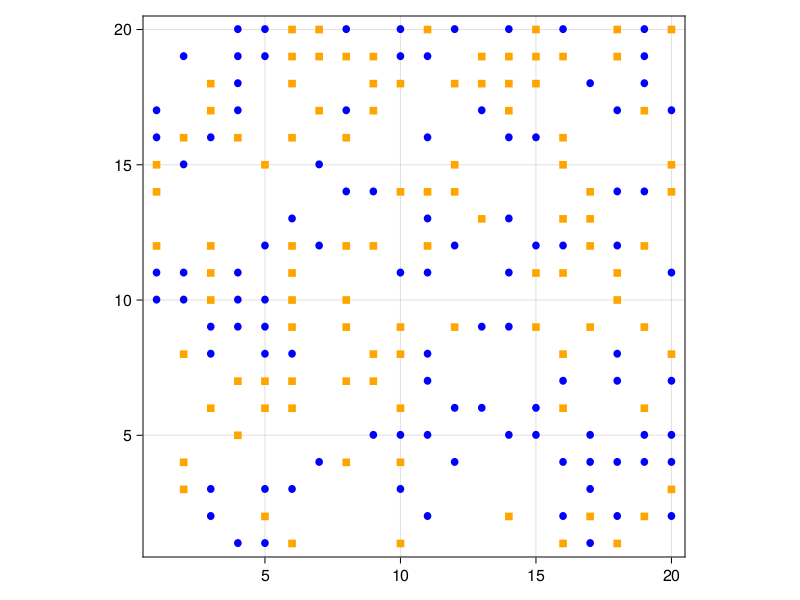Most of the agents have settled happily. Now, let's save the model.

AgentsIO.save_checkpoint("schelling.jld2", model)

Note that we can now leave the REPL, and come back later to run the model, right from where we left off.

model = AgentsIO.load_checkpoint("schelling.jld2"; scheduler = Schedulers.Randomly())

Since functions are not saved, the scheduler has to be passed while loading the model. Let's now verify that we loaded back exactly what we saved.

figure, _ = abmplot(model; ac = groupcolor, am = groupmarker, as = 10)
figureFor starters, let's see what happens if we add 100 more agents of group 1

for i in 1:100
agent = SchellingAgent(nextid(model), (1, 1), false, 1)
end

Let's see what our model looks like now.

figure, _ = abmplot(model; ac = groupcolor, am = groupmarker, as = 10)
figure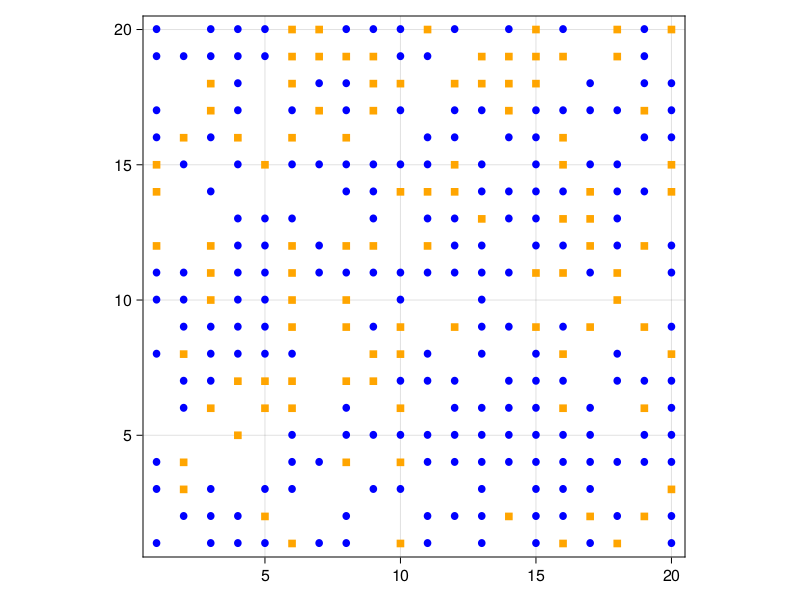And then run it for 40 iterations.

run!(model, agent_step!, 40)

figure, _ = abmplot(model; ac = groupcolor, am = groupmarker, as = 10)
figure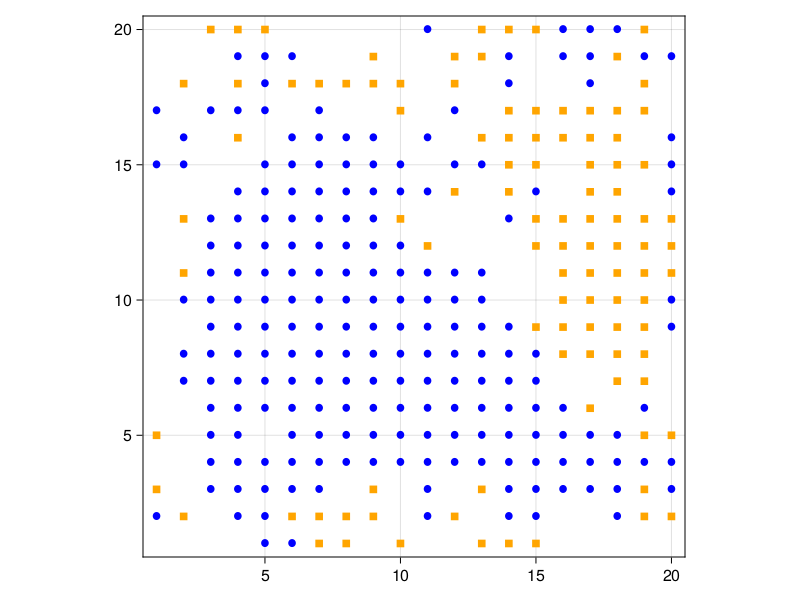It looks like the agents eventually cluster again. What if the agents are of a new group? We can start by loading the model back in from the file, thus resetting the changes we made.

model = AgentsIO.load_checkpoint("schelling.jld2"; scheduler = Schedulers.Randomly())

for i in 1:100
agent = SchellingAgent(nextid(model), (1, 1), false, 3)
end

To visualize the model, we need to redefine groupcolor and groupmarker to handle a third group.

groupcolor(a) = (:blue, :orange, :green)[a.group]
groupmarker(a) = (:circle, :rect, :cross)[a.group]

figure, _ = abmplot(model; ac = groupcolor, am = groupmarker, as = 10)
figureThe new agents are scattered randomly, as expected. Now let's run the model.

run!(model, agent_step!, 40)

figure, _ = abmplot(model; ac = groupcolor, am = groupmarker, as = 10)
figure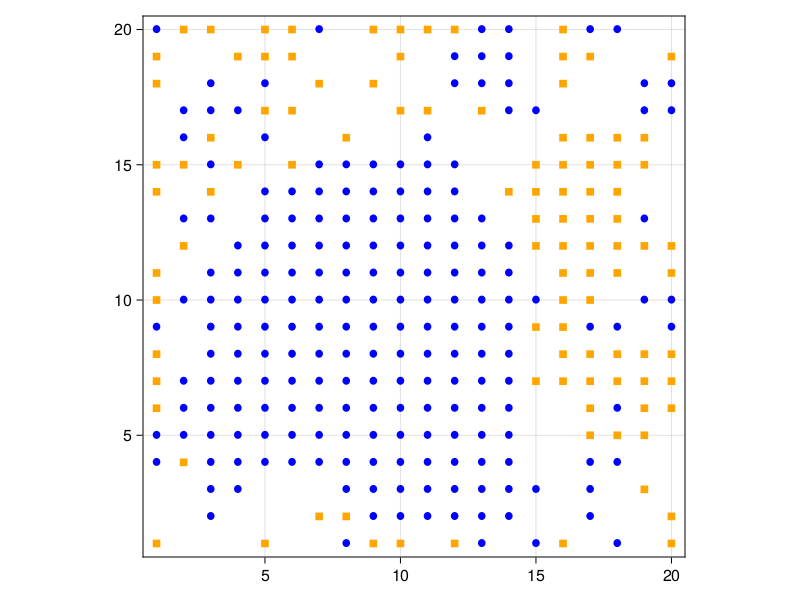The new agents also form their own clusters, despite being completely scattered. It's also interesting to note that there is minimal rearrangement among the existing groups. The new agents simply occupy the remaining space.

## Ensembles and distributed computing

We can run ensemble simulations and collect the output of every member in a single DataFrame. To that end we use the ensemblerun! function. The function accepts a Vector of ABMs, each (typically) initialized with a different seed and/or agent distribution. For example we can do

models = [initialize(seed = x) for x in rand(UInt8, 3)];

and then

adf, = ensemblerun!(models, agent_step!, dummystep, 5; adata)
adf[(end - 10):end, :]
11×4 DataFrame
Rowstepsum_moodmean_xensemble
Int64Int64Float64Int64
1120310.28122
2226110.49382
3328210.50312
4429710.452
5530710.42192
60010.29063
7120010.42813
8225610.39693
9328010.75623
10429510.35943
11530610.63

It is possible to run the ensemble in parallel. For that, we should start julia with julia -p n where n is the number of processing cores. Alternatively, we can define the number of cores from within a Julia session:

using Distributed
addprocs(4)

For distributed computing to work, all definitions must be preceded with @everywhere, e.g.

using Distributed
@everywhere using Agents
@everywhere @agent SchellingAgent ...
@everywhere agent_step!(...) = ...

Then we can tell the ensemblerun! function to run the ensemble in parallel using the keyword parallel = true:

adf, = ensemblerun!(models, agent_step!, dummystep, 5; adata, parallel = true)

## Scanning parameter ranges

We often are interested in the effect of different parameters on the behavior of an agent-based model. Agents.jl provides the function paramscan to automatically explore the effect of different parameter values.

We have already defined our model initialization function as initialize. We now also define a processing function, that returns the percentage of happy agents:

happyperc(moods) = count(moods) / length(moods)

parameters = Dict(
:min_to_be_happy => collect(2:5), # expanded
:numagents => [200, 300],         # expanded
:griddims => (20, 20),            # not Vector = not expanded
)

adf
32×4 DataFrame
Rowstephappyperc_moodmin_to_be_happynumagents
Int64Float64Int64Int64
100.02200
210.5752200
320.8052200
430.8652200
500.03200
610.33200
720.4953200
830.573200
900.04200
1010.144200
1120.2554200
1230.244200
1300.05200
1410.0155200
1520.045200
1630.0355200
1700.02300
1810.8166672300
1920.9266672300
2030.9666672300
2100.03300
2210.5966673300
2320.7533333300
2430.8333333300
2500.04300
2610.354300
2720.4866674300
2830.6633334300
2900.05300
3010.135300
3120.255300
3230.2866675300

We nicely see that the larger :min_to_be_happy is, the slower the convergence to "total happiness".